# Angol nyelvű matematika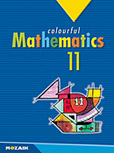## Colourful Mathematics 11.

11. évfolyam, 2. kiadás (2017. 08. 16.)

kód: MS-6311
ára: 4 980 Ft
méret: B5, 296 oldal
Kosárba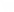TartalomjegyzékMintaoldalakSegédanyag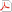Emelt színtű matematika érettségi témakörök (2019)Kapcsolódó kiadványok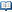MS-6309 - Colourful Mathematics 9.MS-6310 - Colourful Mathematics 10.MS-6312 - Colourful Mathematics 12.MS-2311 - Sokszínű matematika 11.interaktív táblára CLASSROOM Digitális változat elérhetőweb-tankönyv HOME Digitális változat elérhető
 A kiadvány az MS-2311 Sokszínű matematika 11. c. kötet angol nyelvű változata. This book is the English version of the Hungarian market leader textbook titled Sokszínű matematika 11.
 Digitális változat egyedi kóddal*A kiadvány hátsó borítójának belső oldalán található egyedi kóddal a kiadvány digitálisan is elérhető. AKCIÓ! A 2019. augusztus 1. és december 31. között aktivált kódokkal most 4 éves DÍJMENTES hozzáférést biztosítunk a kiadvány mozaWeb Home változatához. A kódok csak egyszer aktiválhatók.
Mintaoldalak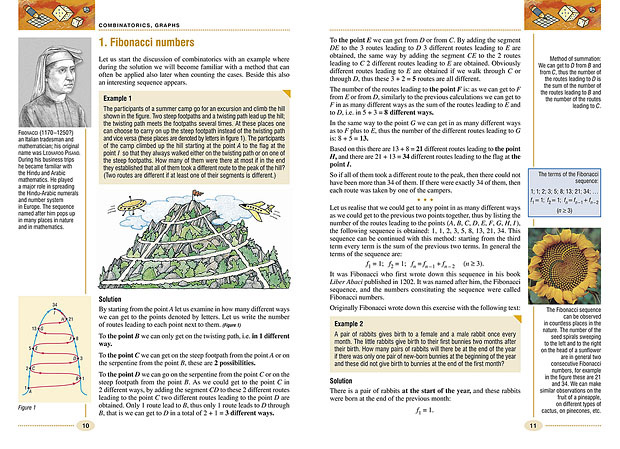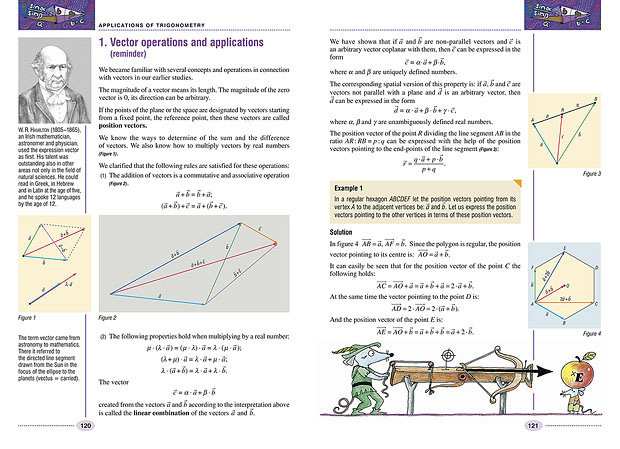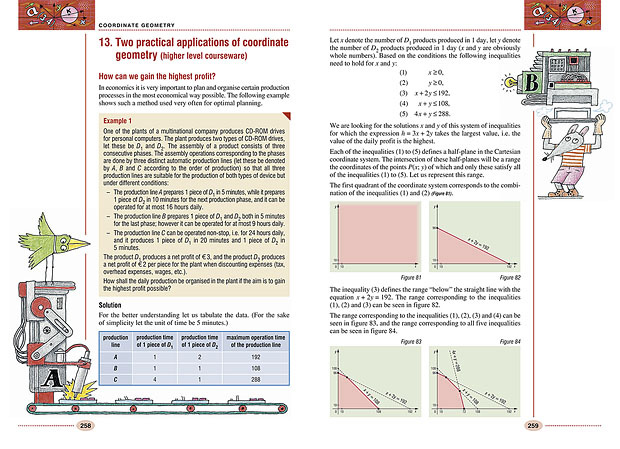Tartalomjegyzék
 Combinatorics, graphs 10 1. Fibonacci numbers 10 2. Permutations, variations . 13 3. Combinations without repetition, Pascal's triangle 20 4. Binomial coefficients, combination with repetition 28 5. Miscellaneous counting exercises (extra-curricular topic) 34 6. GRAPHS - points, edges, degree 38 7. GRAPHS - path, trail, walk, cycle, Eulerian trail (extra-curricular topic) 48 8. Tree graphs (extra-curricular topic) 57 9. Practical applications of combinatorics 63 Powers, roots, logarithms 66 1. Exponentiation and computing the roots (reminder) 66 2. Power functions and root functions 71 3. Power with fractional (rational) exponent 74 4. Power with irrational exponent, exponential function 80 5. Exponential equations, simultaneous exponential equations and exponential inequalities 85 6. The concept of the logarithm 92 7. The logarithm function 98 8. The logarithmic identities 102 9. Logarithmic equations, simultaneous logarithmic equations and logarithmic inequalities . 107 10. Practical applications 115 Applications of trigonometry 120 1. Vector operations, applications (reminder) 120 2. The dot product 125 3. Dot product in the coordinate system 130 4. The sine rule 134 5. The cosine rule 139 6. Applications of the trigonometric relations 143 7. Addition formulae 147 8. Applications of the addition formulae 150 9. Trigonometric equations, inequalities 156 10. The application of trigonometry for positioning 163 Functions 166 1. The exponential and the logarithm function 166 2. Equations and functions 170 3. Trigonometric functions 172 4. Trigonometric equations, inequalities (extra-curricular topic) 176 5. Miscellaneous exercises (extra-curricular topic) 181 6. Inverse functions (extra-curricular topic) 186 7. A practical application of the functions 189 Coordinate geometry 192 1. Vectors in the coordinate system. Operations with vectors given with coordinates (reminder) 192 2. The distance between two points. The angle of inclination of two vectors 195 3. The coordinates of the point of division of a line segment. The coordinates of the centroid of a triangle 198 4. Data defining a straight line in the coordinate system 204 5. The equation of a straight line I. 212 6. The equation of a straight line II. 215 7. The intersection point, the distance and the angle of inclination of two straight lines 219 8. The equation of a circle 225 9. The mutual position of a circle and a straight line; the common points of two circles 233 10. The equation of a parabola 244 11. The parabola and the quadratic function (extra-curricular topic) 250 12. Conic sections and their equations in the coordinate system (extra-curricular topic) 253 13. Two practical applications of coordinate geometry 258 Calculation of probability, statistics 264 1. Classical probability model 264 2. Sampling with replacement 273 3. Sampling without replacement (extra-curricular topic) 280 4. Probabilistic games on graphs (extra-curricular topic) 286 5. Reality and statistics 293